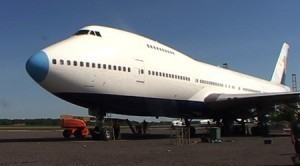•• # Dimensions of a Jumbo Jet

The jumbo jet is a general term used for any type of large aircraft. The following are some of the biggest aircraft ever made.

### Dimensions of a Jumbo Jet: C-5 Galaxy

The length is 247 ft 1 in (75.31 m) and the height is 65 ft 1 in (19.84 m). The payload is 270,000 lb (122,470 kg) and the wingspan is 222 ft 9 in (67.89 m). The wing area is 6,200 ft2 (576 m2). The empty weight is 380,000 lb (172,370 kg) and the loaded weight is 769,000 lb (348,800 kg).

The maximum takeoff weight is 840,000 lb (381,000 kg). The top speed is Mach 0.79 (503 kn / 579 mph, 932 km/h). The cruise speed is Mach 0.77 and the range is 2,400 nmi. The ceiling is 35,700 ft (10,600 m). The rate of climb is 1,800 ft/min (9.14 m/s) and the landing roll is 3,600 ft (1,100 m).

### Dimensions of a Jumbo Jet: Antonov An-124

The Antonov An-124 is 68.96 m (226 ft 3 in) long with a wingspan of 73.3 m (240 ft 5 in). The plane is 20.78 m (68 ft 2 in high. The payload is 150,000 kg (330,000 lb). The wing area is 628 m2 (6,760 sq ft). The empty weight is 175,000 kg (385,000 lb), while the loaded weight is 405,000 kg (893,000 lb).

The takeoff weight limit is 405,000 kg (893,000 lb). The maximum speed is 865 km/h (467 kn / 537 mph). The cruise speed is 800-850 km/h (430 kn / 490 mph). The service ceiling is 12,000 m (35,000 ft). The wing loading is 365 kg/m2 (74.7 lb/sq ft) and the thrust/weight is 0.41.

### Dimensions of a Jumbo Jet: 747-300

The 747-300 is 231 ft 10 in (70.6 m) long with a wingspan of 195 ft 8 in (59.6 m). The tail height is 63 ft 5 in (19.3 m). The empty weight is 392,800 lb (78,100 kg). The maximum takeoff weight is 833,000 lb (377,842 kg).

The cruising speed is Mach 0.84 (893 km/h, 555 mph). The top speed is Mach 0.89 (955 km/h). The maximum range is 6,700 nmi (12,400 km). The fuel capacity is 52,410 U.S. gal. The cockpit crew is three, with a seating capacity of 496 (2-class) / 412 (3-class).

### Dimensions of a Jumbo Jet: Shuttle Carrier Aircraft

The Shuttle Carrier Aircraft is 231 ft 4 in (70.5 m) and the wingspan is 195 ft 8 in (59.7 m). The wing area is 5,500 ft2 (510 m2). The height is 63 ft 5 in (19.3 m). The maximum takeoff weight is 710,000 lb (322,000 kg) while the empty weight is 318,000 lb (144,200 kg).

The cruising speed is Mach 0.6 (735 km/h, 457 mph). The range is 1,150 mi (1,000 nmi, 1,850 km) and the service ceiling is 15,000 ft (4,500 m). The crew is four (pilot, co-pilot and two flight engineers).

The dimensions of a jumbo jet continue to expand as demand for comfortable air travel increases. Along with an increase in physical size, safety features are implemented in new models.